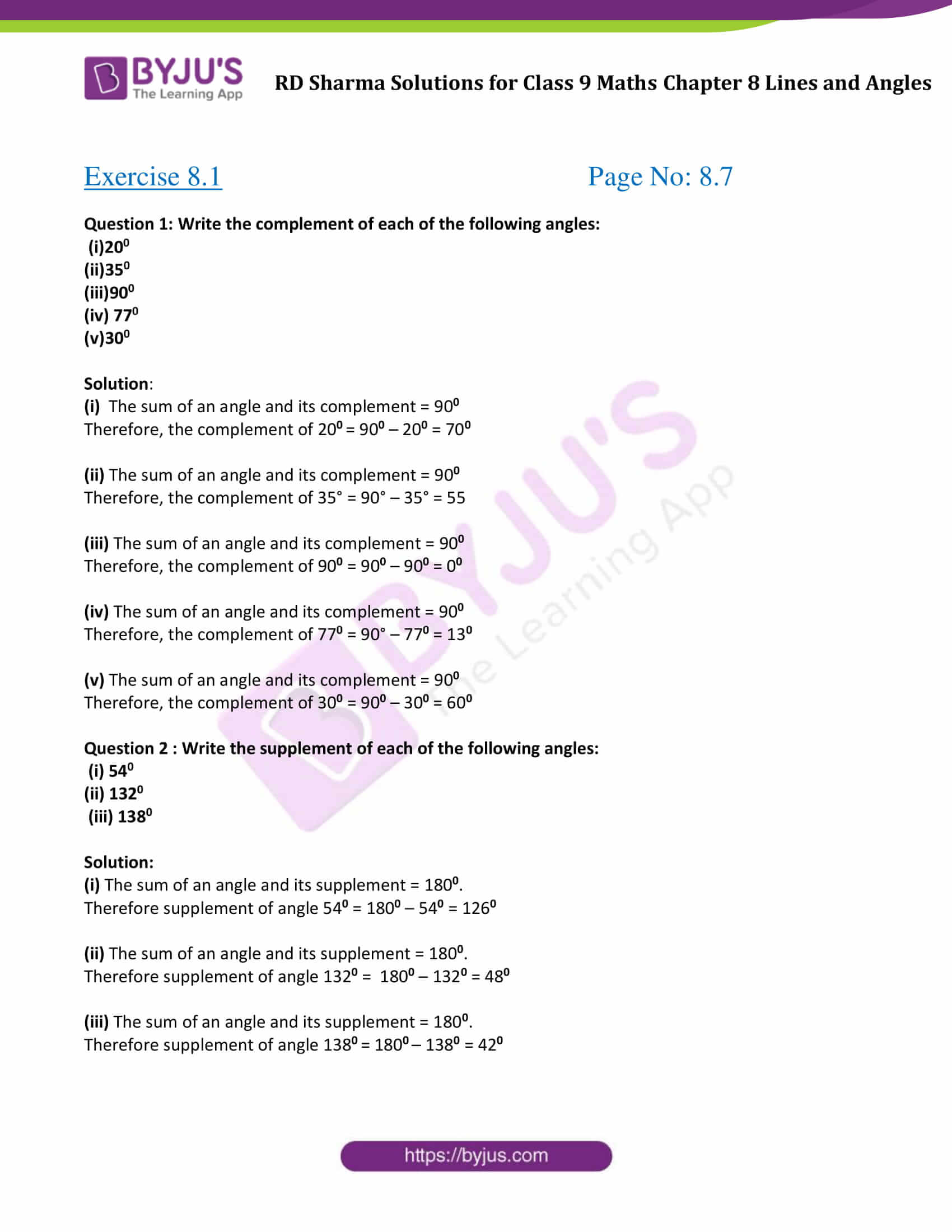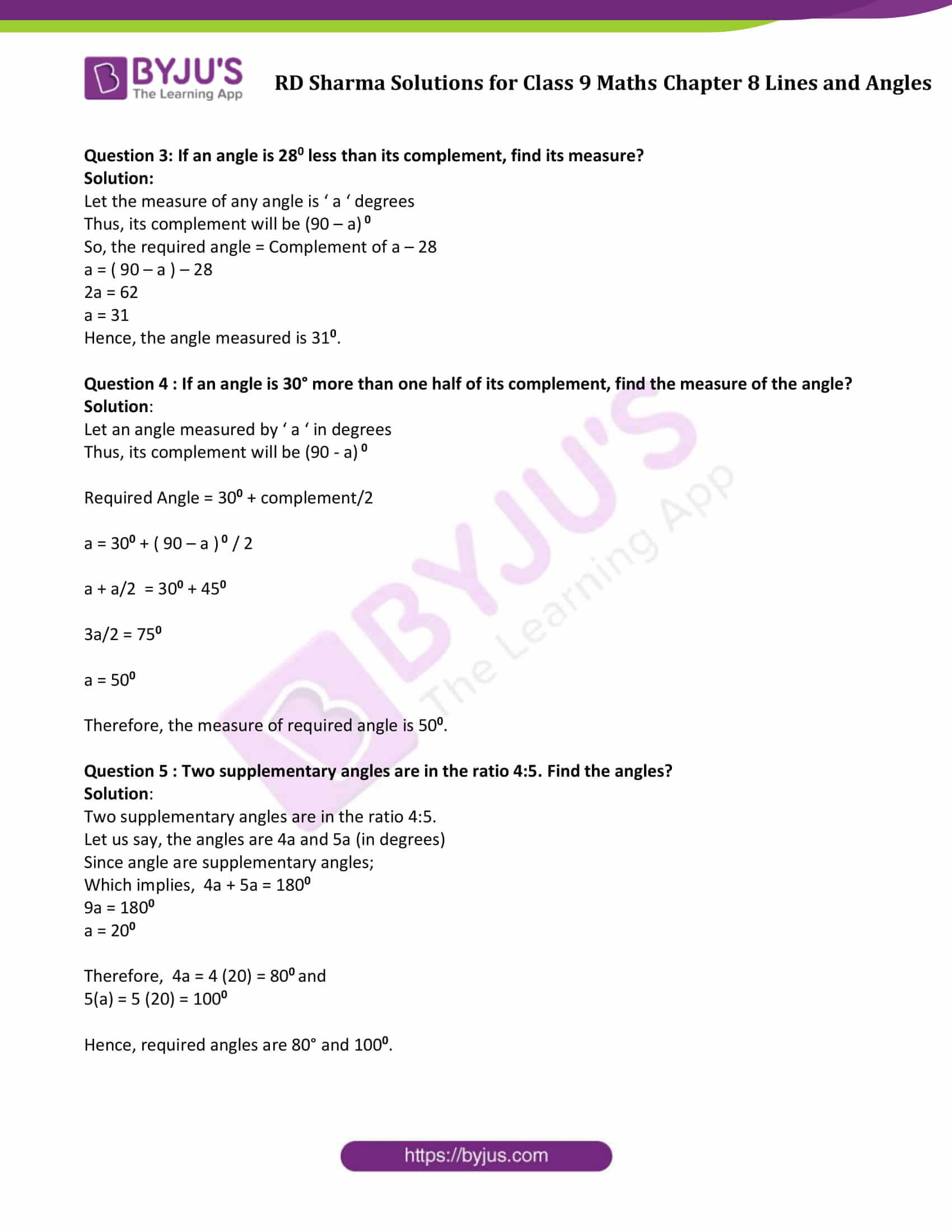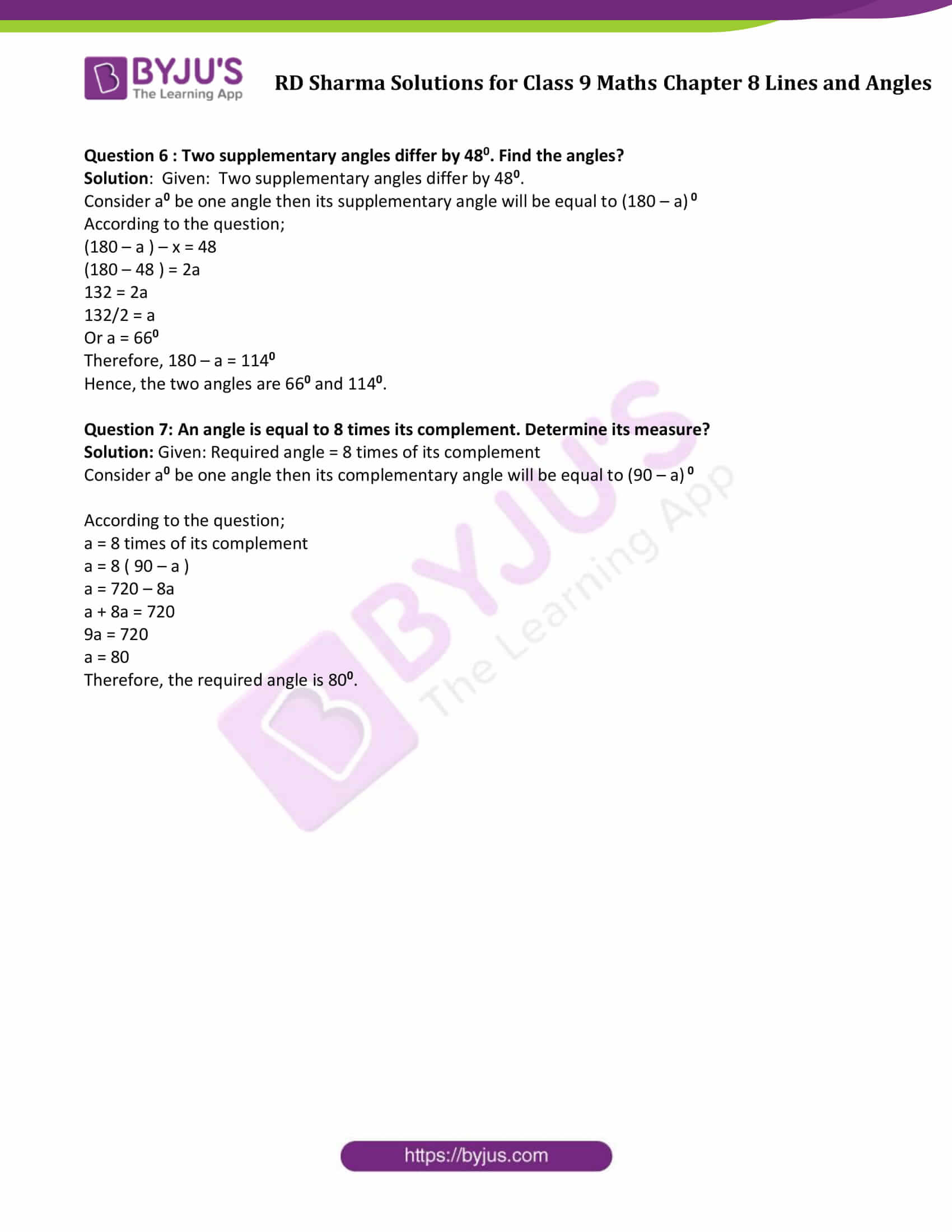# RD Sharma Solutions for Class 9 Maths Chapter 8 Lines and Angles Exercise 8.1

RD Sharma Solutions for Class 9 Maths are given here for Chapter 8 – Lines and Angles. In order to make concepts easier for students, we are providing RD Sharma Class 9 Maths Chapter 8 solutions to help them go through several solved exercises and at the same time practice the questions. The RD Sharma solutions for class 9 can help students discover new ways to solve difficult problems. Students can download RD Sharma Class 9 solutions and practice offline.

## Download PDF of RD Sharma Solutions for Class 9 Maths Chapter 8 Lines and Angles Exercise 8.1### Access Answers to Maths RD Sharma Solutions for Class 9 Chapter 8 Lines and Angles Exercise 8.1 Page number 8.7

Question 1: Write the complement of each of the following angles:

(i)200

(ii)350

(iii)900

(iv) 770

(v)300

Solution:

(i) The sum of an angle and its complement = 900

Therefore, the complement of 200 = 900 – 200 = 700

(ii) The sum of an angle and its complement = 900

Therefore, the complement of 35° = 90° – 35° = 55

(iii) The sum of an angle and its complement = 900

Therefore, the complement of 900 = 900 – 900 = 00

(iv) The sum of an angle and its complement = 900

Therefore, the complement of 770 = 90° – 770 = 130

(v) The sum of an angle and its complement = 900

Therefore, the complement of 300 = 900 – 300 = 600

Question 2 : Write the supplement of each of the following angles:

(i) 540

(ii) 1320

(iii) 1380

Solution:

(i) The sum of an angle and its supplement = 1800.

Therefore supplement of angle 540 = 1800 – 540 = 1260

(ii) The sum of an angle and its supplement = 1800.

Therefore supplement of angle 1320 = 1800 – 1320 = 480

(iii) The sum of an angle and its supplement = 1800.

Therefore supplement of angle 1380 = 1800 – 1380 = 420

Question 3: If an angle is 280 less than its complement, find its measure?

Solution:

Let the measure of any angle is ‘ a ‘ degrees

Thus, its complement will be (90 – a) 0

So, the required angle = Complement of a – 28

a = ( 90 – a ) – 28

2a = 62

a = 31

Hence, the angle measured is 310.

Question 4 : If an angle is 30° more than one half of its complement, find the measure of the angle?

Solution:

Let an angle measured by ‘ a ‘ in degrees

Thus, its complement will be (90 – a) 0

Required Angle = 300 + complement/2

a = 300 + ( 90 – a ) 0 / 2

a + a/2 = 300 + 450

3a/2 = 750

a = 500

Therefore, the measure of required angle is 500.

Question 5 : Two supplementary angles are in the ratio 4:5. Find the angles?

Solution:

Two supplementary angles are in the ratio 4:5.

Let us say, the angles are 4a and 5a (in degrees)

Since angle are supplementary angles;

Which implies, 4a + 5a = 1800

9a = 1800

a = 200

Therefore, 4a = 4 (20) = 800 and

5(a) = 5 (20) = 1000

Hence, required angles are 80° and 1000.

Question 6 : Two supplementary angles differ by 480. Find the angles?

Solution: Given: Two supplementary angles differ by 480.

Consider a0 be one angle then its supplementary angle will be equal to (180 – a) 0

According to the question;

(180 – a ) – x = 48

(180 – 48 ) = 2a

132 = 2a

132/2 = a

Or a = 660

Therefore, 180 – a = 1140

Hence, the two angles are 660 and 1140.

Question 7: An angle is equal to 8 times its complement. Determine its measure?

Solution: Given: Required angle = 8 times of its complement

Consider a0 be one angle then its complementary angle will be equal to (90 – a) 0

According to the question;

a = 8 times of its complement

a = 8 ( 90 – a )

a = 720 – 8a

a + 8a = 720

9a = 720

a = 80

Therefore, the required angle is 800.

## RD Sharma Solutions for Class 9 Maths Chapter 8 Lines and Angles Exercise 8.1

Class 9 Maths Chapter 8 Lines and Angles Exercise 8.1 is based on the following topics and subtopics:

• Angles
• Interior of an angle
• Exterior of an angle
• Congruent angles
• Measure of an angle
• Axioms and Theorems
• Types of angles
• -Right Angle
• -Acute Angle
• -Obtuse Angle
• -Straight Angle
• -Reflex Angle
• -Complementary Angles
• -Supplementary Angles
• Angle Bisector